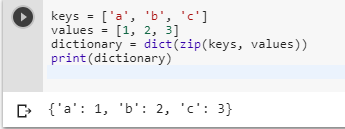2 views
in Python

Imagine that you have:

keys = ['name', 'age', 'food'] values = ['Monty', 42, 'spam']

What is the simplest way to produce the following dictionary?

a_dict = {'name' : 'Monty', 'age' : 42, 'food' : 'spam'}

by (106k points)

To convert list values into dictionary the pairwise dict constructor and zip function are awesomely useful:

dict():-

The dict() constructor creates dictionaries directly from lists of key-value pairs.

zip():-

The zip() function in Python returns a zip object, which is an iterator of list/tuple where the first item in each passed iterator is paired together, and then the second item in each passed iterator are paired together, etc.

The following code is an example that solves your problem:-

keys = ['a', 'b', 'c']

values = [1, 2, 3]

dictionary = dict(zip(keys, values))

print(dictionary)